# WebPlatform Documentation

## Appendix: Calculated Data Formats

### What you need to know about Calculated data

Field type "Calculated Data" accepts formatting information using standard formatting (Standard Numeric Format Strings):

https://msdn.microsoft.com/en-us/library/427bttx3.aspx

Data will be displayed in all forms according to the entered formatting information

• Especially %

If no formatting information is present, the default (present) format is used

Note: Formatting applies to the display to users, not the underlying data in the field. So for reporting purposes, the value in the cell will be unchanged. Thus reporting is unaffected by this functionality

### How to use Calculated Data Standard Formats

• If you choose to apply a format to your calculated data value, these are the selectable options: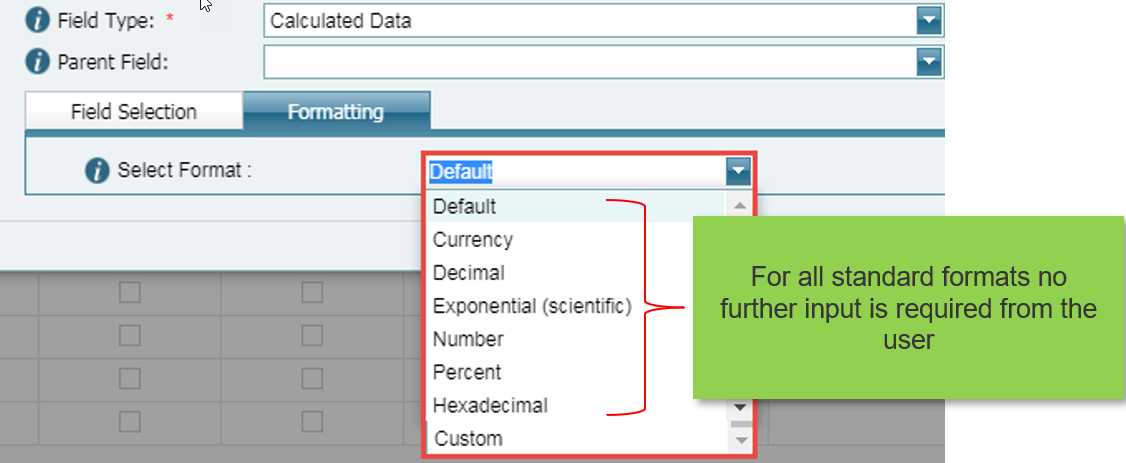### What to expect for Default format

• Applies if Default is selected for formatting or if left blank
• Default format will display the same as the source field configuration
• i.e. The exact result of the calculation (without any symbol or placers)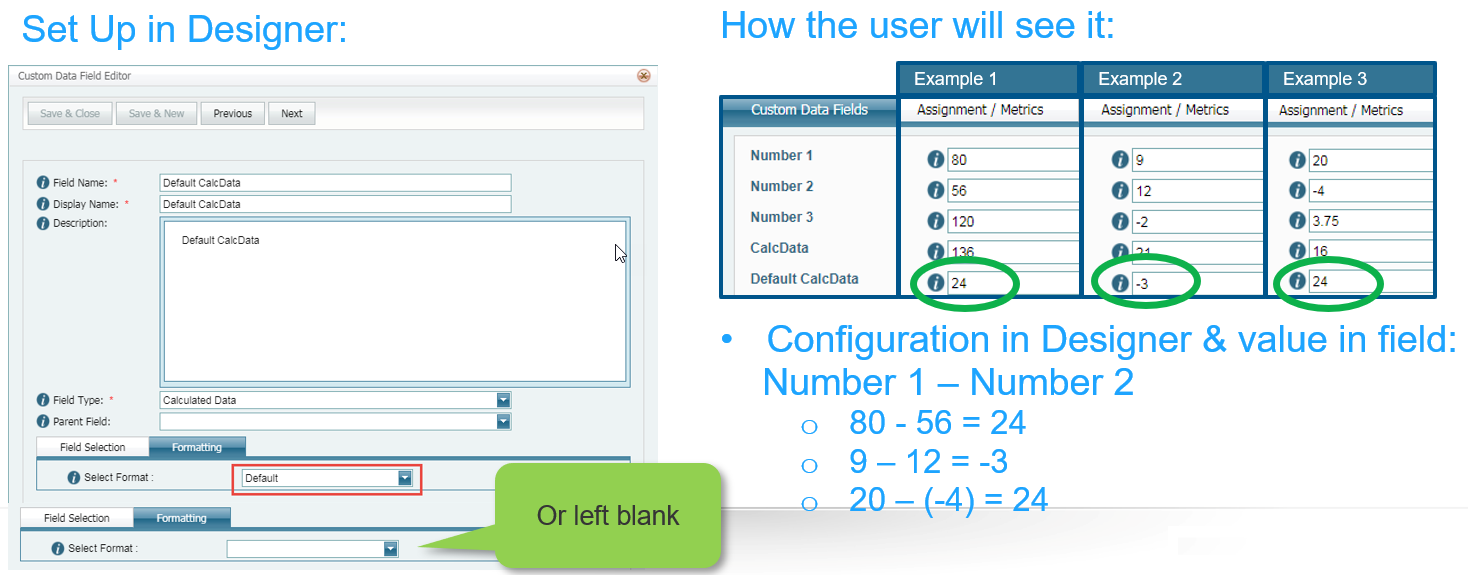### What to expect for Currency format

• A currency value as per the set up
• i.e. The exact result of the calculation using current culture* symbol = \$### What to expect for Number format

• Integral and decimal digits, group separators, and a decimal separator with optional negative sign
• i.e. Standard Number = number with 2 decimal spaces### What to expect for Percent format

• Number multiplied by 100 and displayed with a percent symbol
• i.e. Standard Percent always rounded to 2 decimal places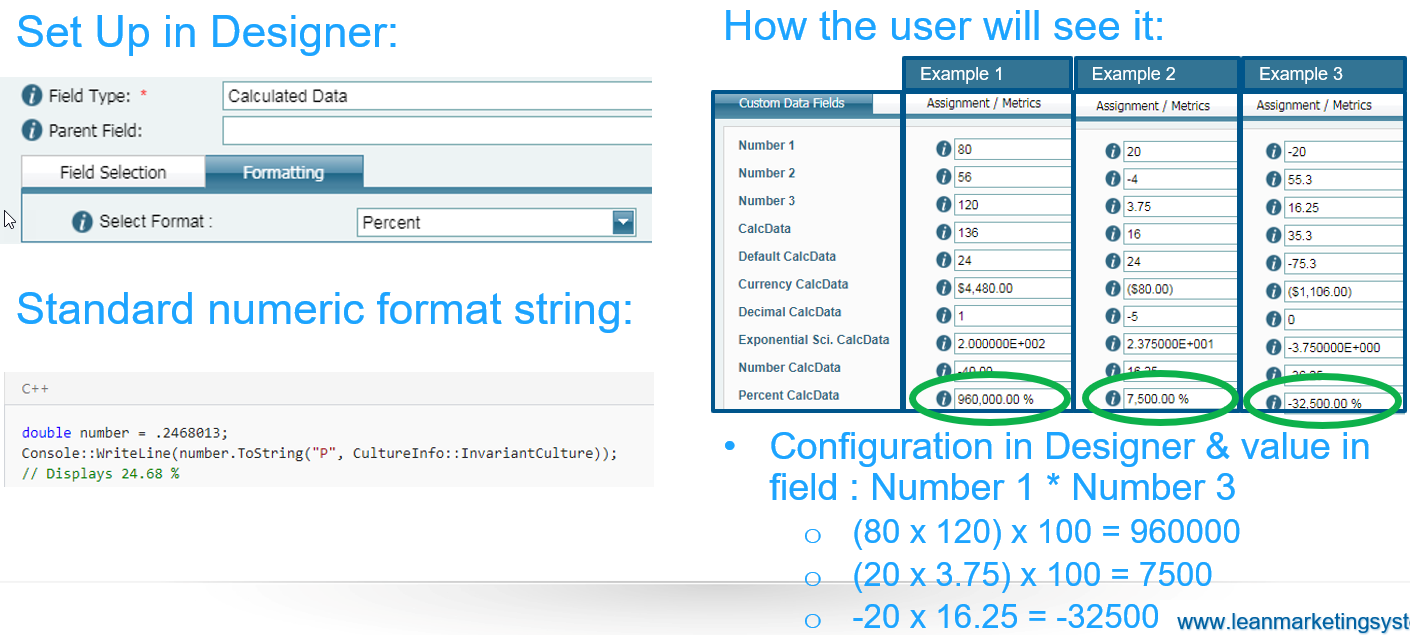### How to use the percent format correctly (Example for Percent Format)

• If you want to display a calculation as if you were doing it on a calculator, all you need to do is format the final calculation to be displayed in format = Percent
• Here’s an example of how this new functionality will work for eLearning processes

Step 1: Modify Fixed list of values & descriptions to have the score in value column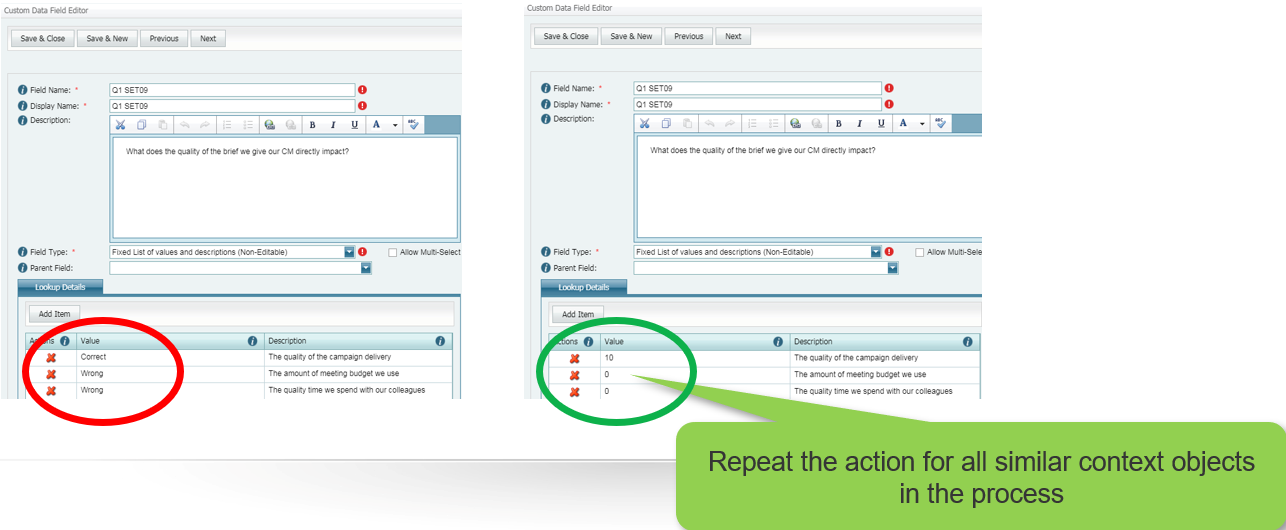Step 2: Add calculation for all scores achieved (in this example there are 7 questions, so add all fields)

• Do not apply Formatting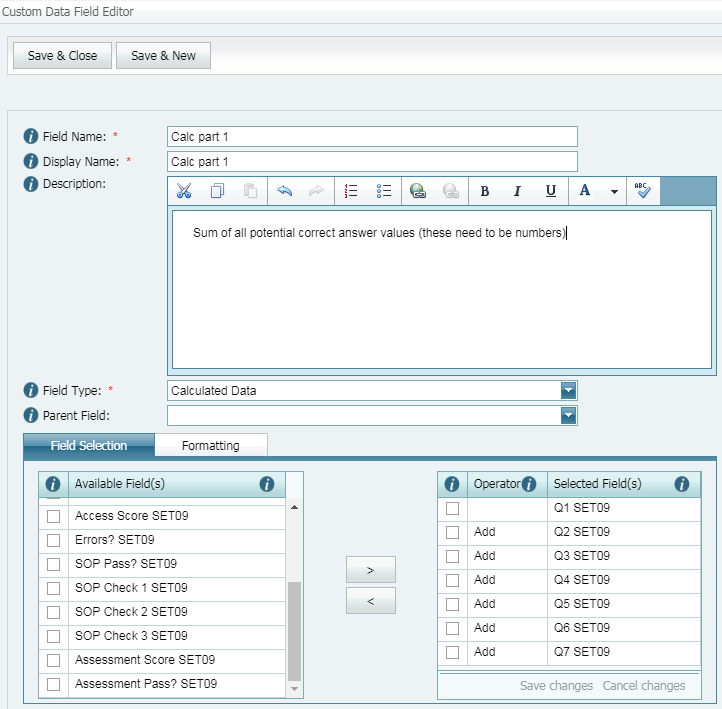Step 3: Add new number context object for maximum achievable score value (for 100% achievement)Step 4: Add the second calculation

• Achieved / Maximum achievableIn the Formatting tab

• Select Formatting = Percent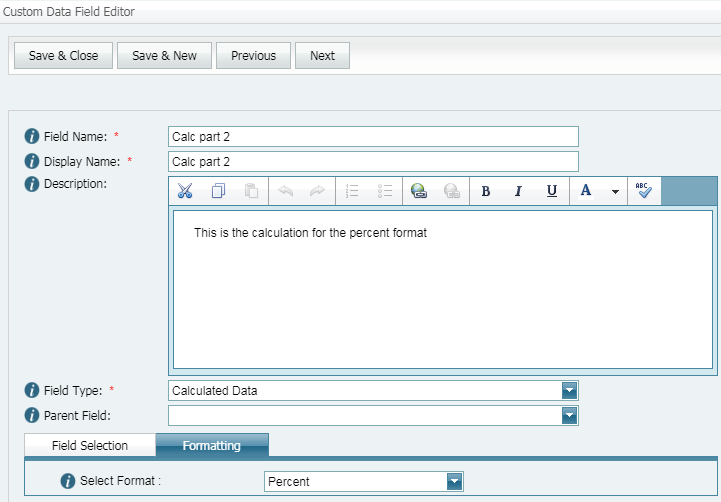Step 5: Update forms

• Before and after example### This is what the user will experience

• Instead of correct/wrong answers, the user marking the test will see the number of points achieved for each answer
• As the module score is a calculation it will be read-only
• by having this done automatically we eliminate human error### What to expect for Hexadecimal format

• A hexadecimal string for Integral types only (integers)
• Where to check hexadecimal conversions: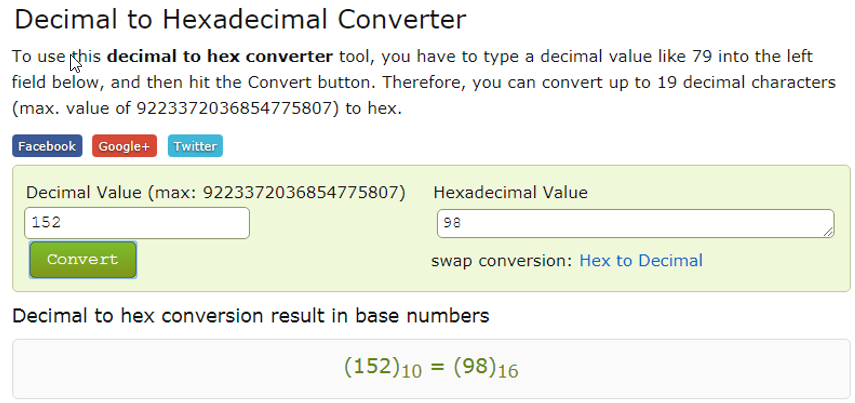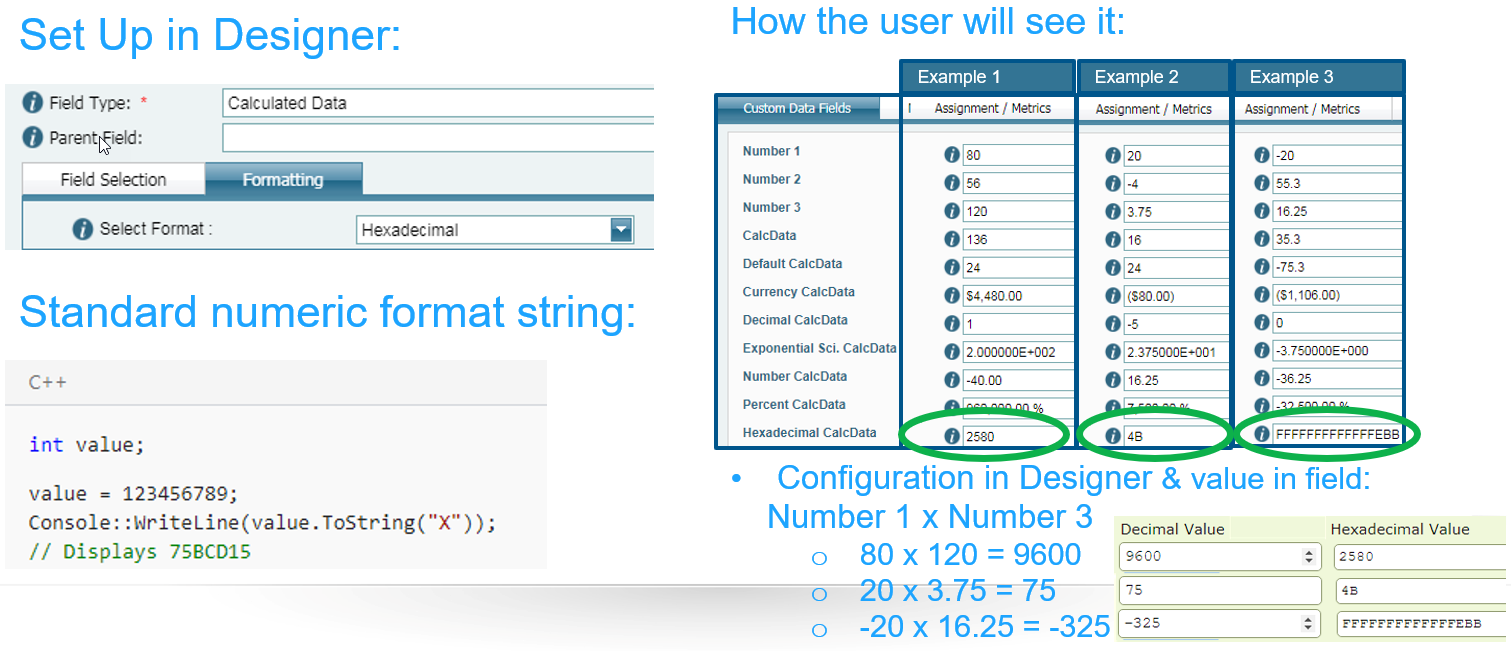### What to expect for Decimal format

• Integer digits with optional negative sign
• i.e. The result of the calculation narrowed down to the integer digit only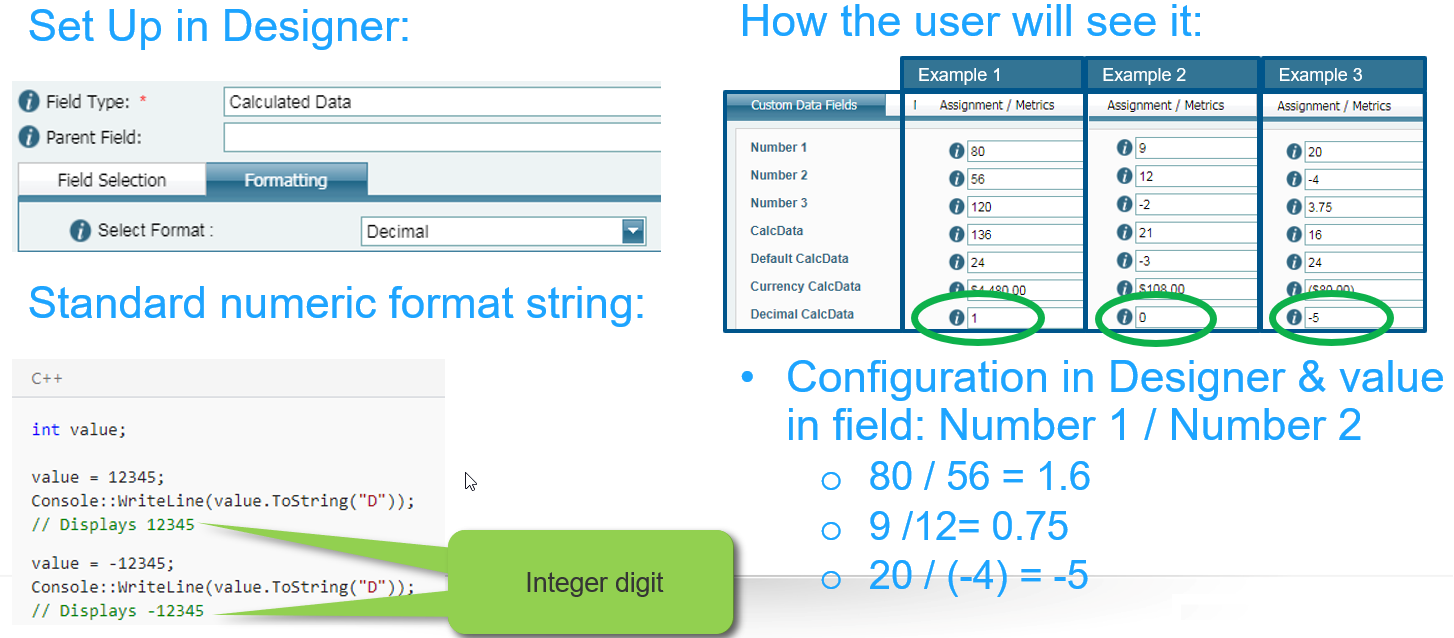### What to expect for Exponential (scientific) format

• Exponential notation
• i.e. The result of the calculation narrowed down to the integer digit only### Customise in Formatting tab for field type = Calculated Data

• You choose to apply a ‘Custom’ format to your calculated data value, a new field will load where you can add the string you want to use:### What to expect for Zero placeholder format

• Replaces the zero with the corresponding digit if one is present; otherwise, zero appears in the result string.
• To five digits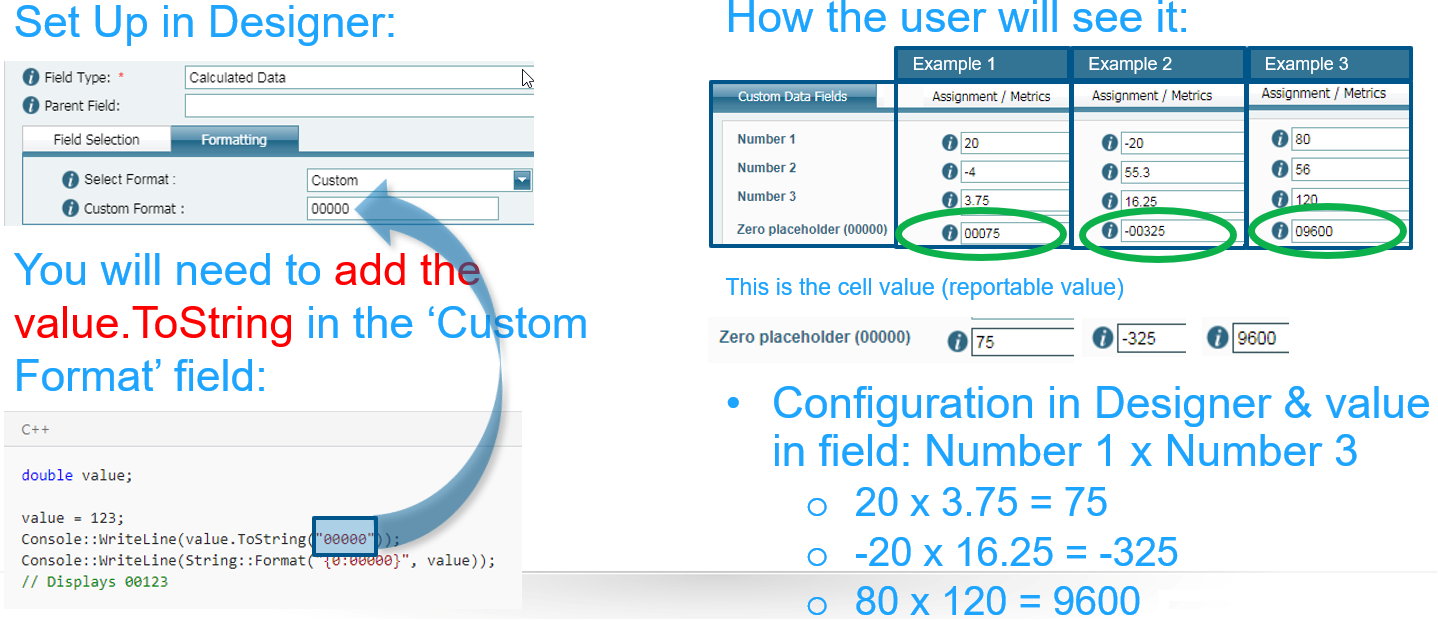### What to expect for Digit placeholder format

• Replaces the "#" symbol with the corresponding digit if one is present; otherwise, no digit appears in the result string.
• Note that no digit appears in the result string if the corresponding digit in the input string is a non-significant 0. For example, 0003 ("####") -> 3.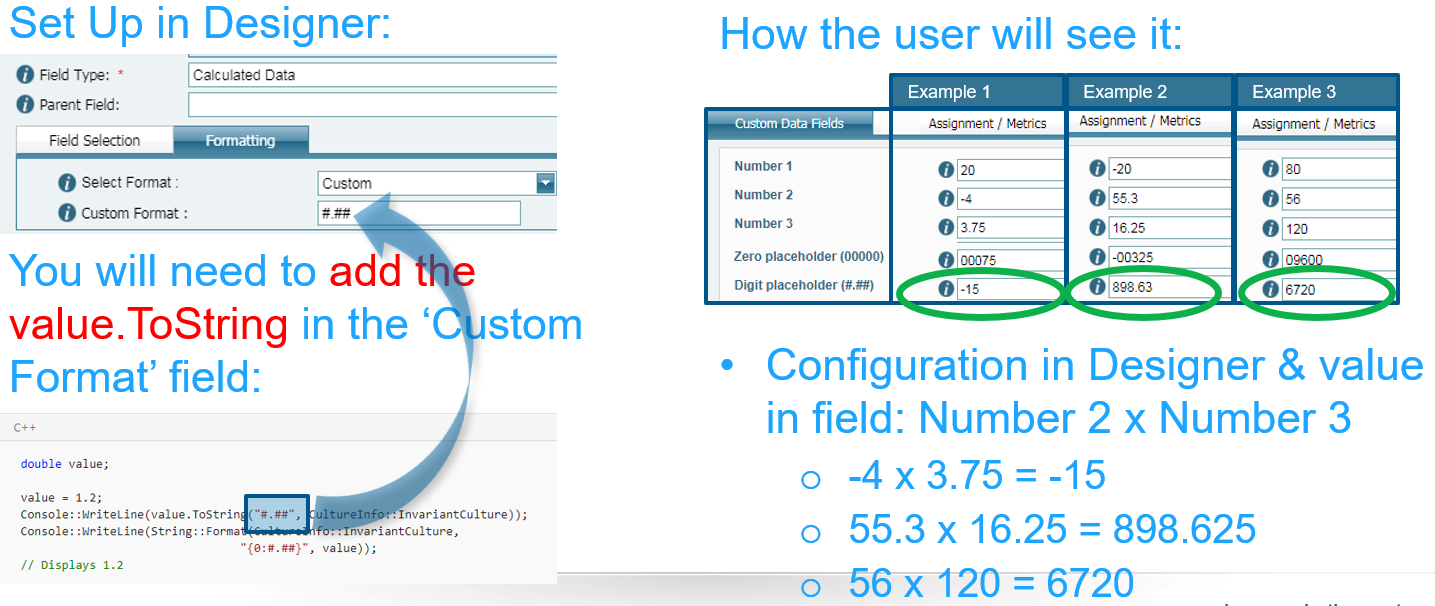### What to expect for Decimal point format

• Determines the location of the decimal separator in the result string.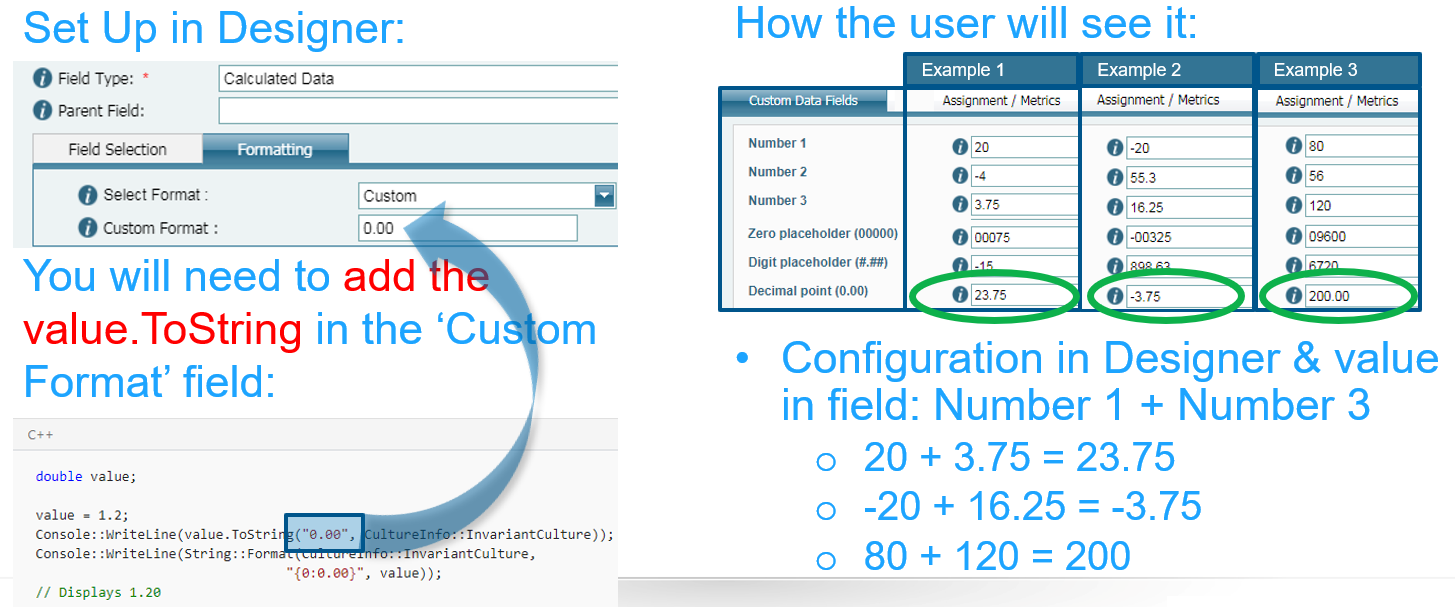### What to expect for Group Separator format

• Serves as both a group separator and a number scaling specifier. As a group separator, it inserts a localized group separator character between each group.
• As a number scaling specifier, it divides a number by 1000 for each comma specified.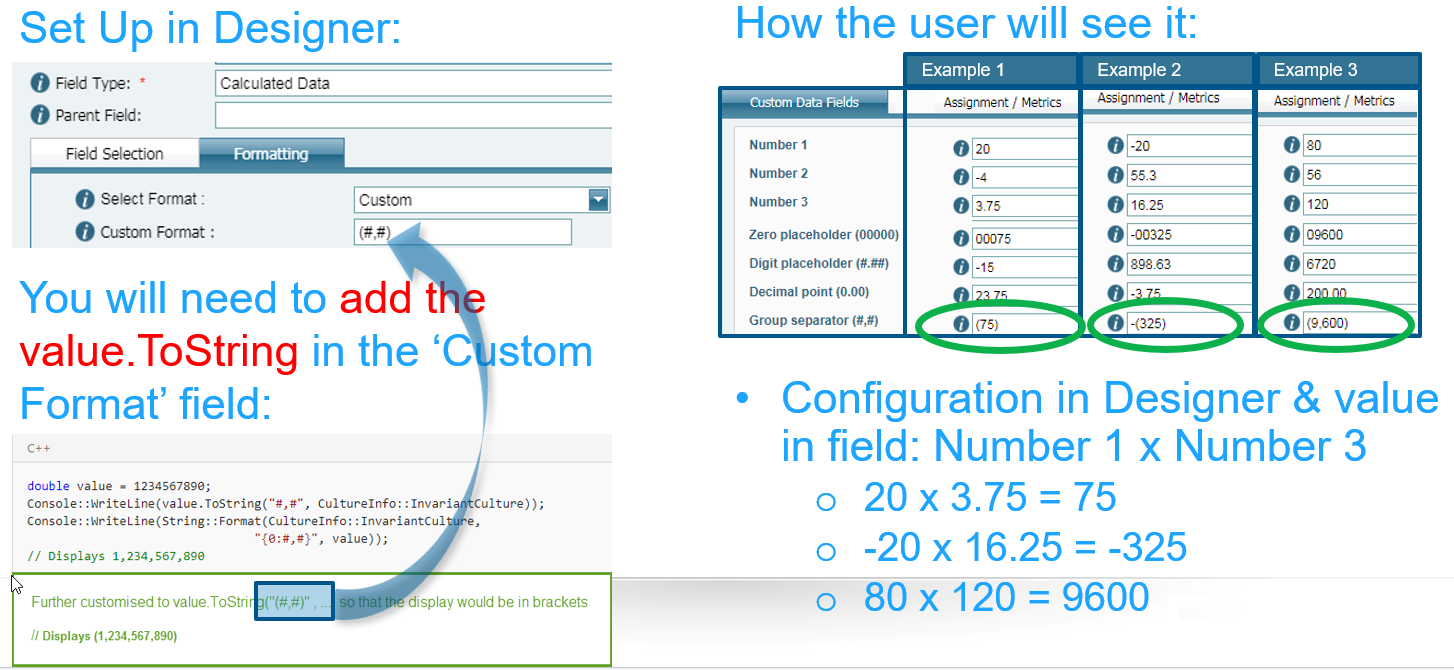### What to expect for Exponential Notation format

• If followed by at least one 0 (zero), formats the result using exponential notation. The case of "E" or "e" indicates the case of the exponent symbol in the result string. The number of zeros following the "E" or "e" character determines the minimum number of digits in the exponent. A plus sign (+) indicates that a sign character always precedes the exponent. A minus sign (-) indicates that a sign character precedes only negative exponents.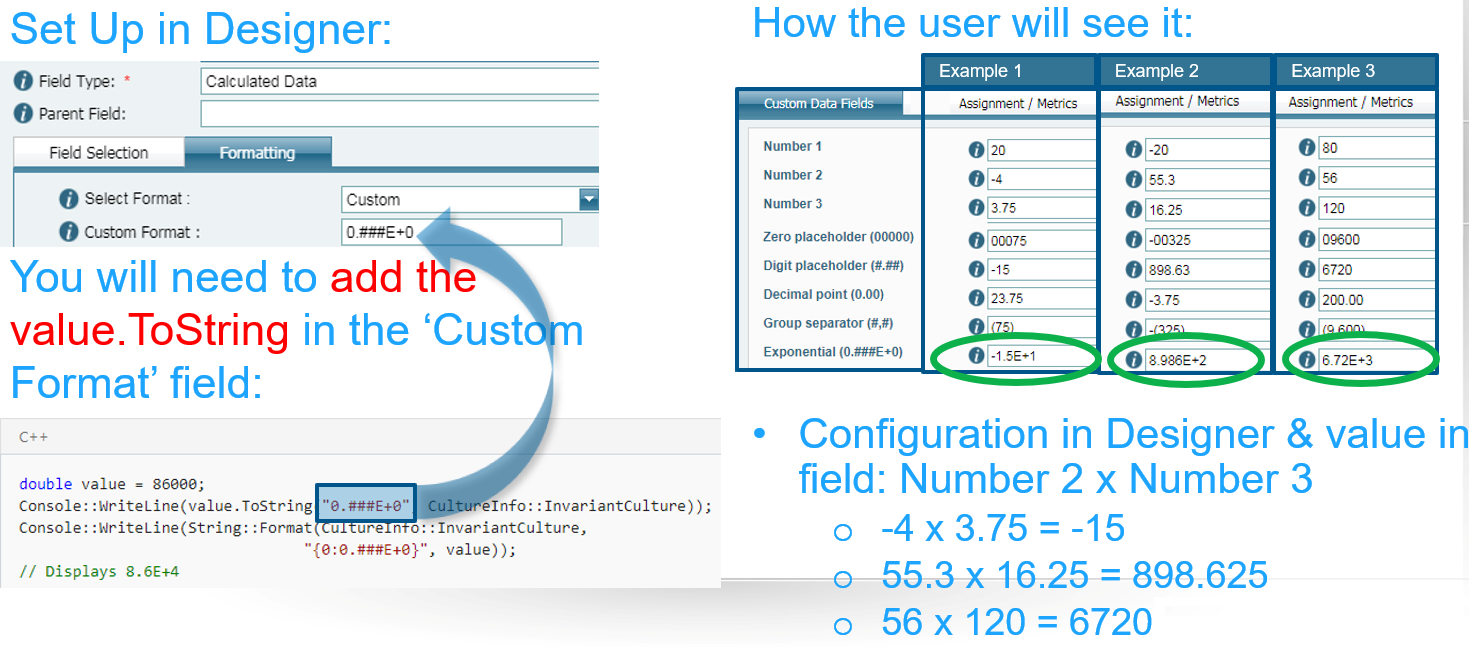### What to expect for Per Mille format

• Multiplies a number by 1000 and inserts a localized per mille symbol in the result string.help_outline